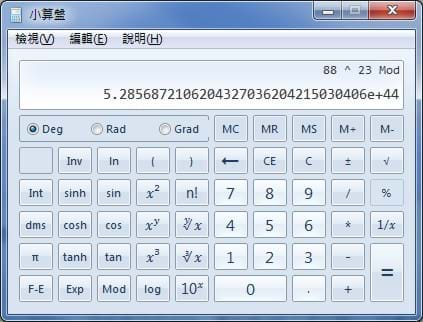﻿

# RSA公鑰與私鑰運算之演算過程

## RSA源起``````1230186684530117755130494958384962720772853569595334792197
3224521517264005072636575187452021997864693899564749427740
6384592519255732630345373154826850791702612214291346167042
9214311602221240479274737794080665351419597459856902143413
=
3347807169895689878604416984821269081770479498371376856891
2431388982883793878002287614711652531743087737814467999489
×
3674604366679959042824463379962795263227915816434308764267
6032283815739666511279233373417143396810270092798736308917
``````

## 歐拉函數

RSA運算的核心起源於歐拉函數，歐拉函數的定義是在數論中，對正整數n，小於或等於n的正整數中與n互質的總個數（互質的定義請參考WiKi 互質）。此函數以其首名研究者歐拉命名，它又稱為φ函數。舉例來說：

``````φ(8) = 4; 因為1, 3, 5, 7均和8互質。
``````

``````n = p \* q;  //令p與q互質
φ(n) = φ(p\*q) = φ(p)\*φ(q) = (p-1)\*(q-1);
``````

``````n = 11 \* 13 = 143;
φ(143) = φ(11\*13) = φ(11)\*φ(13) = (11-1)\*(13-1) = 120;
``````

## 模反元素

``````ed ≡ 1 (mod φ(n))
``````

``````Extended Euclidean Algorithm Strat
--------------
47X + 30Y =  1 (舉用WiKi - http://goo.gl/WQij6F的例子)
--------------

--------------
47 = 1 \* 30 + 17
30 = 1 \* 17 + 13
17 = 1 \* 13 +  4
13 = 3 \*  4 +  1
--------------

--------------
47 - 1 \* 30 = 17
30 - 1 \* 17 = 13
17 - 1 \* 13 =  4
13 - 3 \*  4 =  1
--------------

--------------
1 \* 13 - 3 \*  4 =  1 (抄上面最後一行)
1 \* 13 - 3 \* (17 - 1 \* 13) = 1 (記住一個觀念，千萬不可以把項式乘開)
1 \* 13 - 3 \* 17 + (3 \* 13) = 1
-3 \* 17 + 4 \* 13 = 1
-3 \* 17 + 4 \* (30 - 1 \* 17) = 1
-3 \* 17 + (4 \* 30) - (4 \* 17) = 1
4 \* 30 - (7 \* 17) = 1
4 \* 30 - 7 \* (47 - 1 \* 30) = 1
4 \* 30 - (7 \* 47) + (7 \* 30) = 1
-7 \* 47 + (11 \* 30) = 1
--------------

--------------

--------------

23X + 120Y = 1
--------------
120 = 5 \* 23 + 5
23 = 4 \*  5 + 3
5 = 1 \*  3 + 2
3 = 1 \*  2 + 1
--------------

--------------
120 - 5 \* 23 = 5
23 - 4 \*  5 = 3
5 - 1 \*  3 = 2
3 - 1 \*  2 = 1
--------------

--------------
1 \*  3 - 1 \* 2 = 1
1 \*  3 - 1 \* (5 - 1 \*  3) = 1
1 \*  3 - 1 \* 5 + 1 \* 3 = 1
-1 \*  5 + 2 \* 3 = 1
-1 \*  5 + 2 \* (23 - 4 \*  5) = 1
-1 \*  5 + 2 \* 23 - 8 \* 5 = 1
2 \* 23 - 9 \* 5 = 1
2 \* 23 - 9 \* (120 - 5 \* 23) = 1
2 \* 23 - 9 \* 120 + 45 \* 23 = 1
47 \* 23 - 9 \* 120 = 1
--------------

--------------
``````

## 公鑰與私鑰的封裝

``````p = 11 （產生完馬上丟棄）
q = 13 （產生完馬上丟棄）
n = p \* q = 143
φ(n) = 120
e = 23  //encode
d = 27  //decode

``````

## 公鑰與私鑰的驗證

``````因為 m^e ≡ c (mod n) //message，而X的ASCII是88（10進制）
88^23 ≡ c (mod 143)
c = 121  //RSA加密後的文字
``````

P.S Windows 7之後的小算盤可以開起工程模式進行計算，千萬不要笨到自己用手去算88的23次方，除非你很無聊，詳見下圖。另外我們也可以在圖中發現與明白，我們用超簡單的兩位數質數產生的運算就有多龐大了，可見RSA演算法消耗的CPU運算是很恐怖的。A收到由B送過來的資料「121」，取出自己的私鑰(143, 47)，套用RSA的解密公式進行運算「c^d ≡ m (mod n)」。

``````因為 c^d ≡ m (mod n) //content
121^47 ≡ c (mod 143)
c = 88  //RSA解密後的文字，即為X的ASCII碼88（10進制），故證明之。
``````

### 寫在最後

``````RSA-1024 =
135066410865995223349603216278805969938881475605667027524485143851526510604859533833940287150571909441798207282164471551373680419703964191743046496589274256239341020864383202110372958725762358509643110564073501508187510676594629205563685529475213500852879416377328533906109750544334999811150056977236890927563
``````